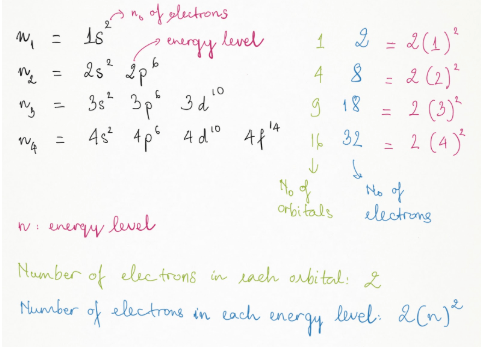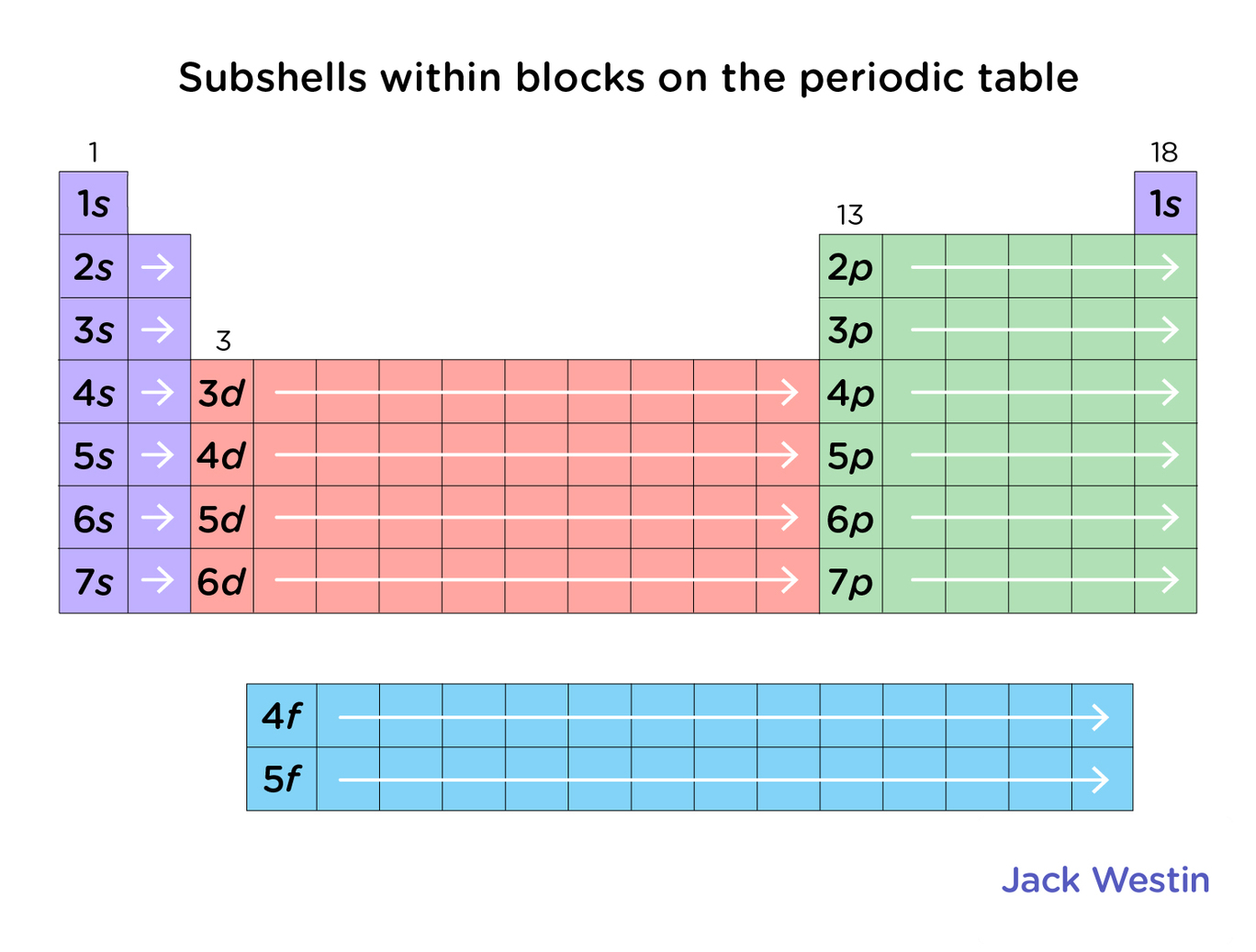Cyber Sale - Only 3 Days Remaining: ALL MCAT Products & Admissions Services

MCAT Content / Electronic Structure / Orbital Structure Of Hydrogen Atom Principal Quantum Number N Number Of Electrons Per Orbital

### Orbital structure of hydrogen atom, principal quantum number n, number of electrons per orbital

Topic: Electronic Structure

A hydrogen atom is electrically neutral, containing a single proton and a single electron bound to the nucleus by the Coulomb force.

Atomic hydrogen comprises about 75 per cent of the elemental mass of the universe. Hydrogen atoms are so reactive that they combine with almost all elements. Isolated hydrogen atoms called atomic hydrogen or monatomic hydrogen) are extremely rare; hydrogen is more commonly found in combination with other elements or its elemental diatomic form H2. The H–H bond is one of the strongest bonds in chemistry.

An orbital can be understood as the physical region or space where the electron is likely to be present. Quantum numbers provide a numerical description of the orbitals in which electrons reside. To describe an electron, we use four quantum numbers are needed: energy (n), angular momentum (ℓ), magnetic moment (m), and spin (s). Hydrogen can be used to model this as it contains 1 electron in 1 orbital.

Each such orbital can be occupied by a maximum of two electrons, each with its spin quantum number (s). The first quantum number n, describes the electron shell, or energy level, of an atom. The value of n ranges from 1 to the shell containing the outermost electron of that atom. The simple names s orbital, p orbital, d orbital, and f orbital refer to orbitals with angular momentum quantum number ℓ = 0, 1, 2, and 3 respectively.

If atoms are isoelectronic they are said to have the same number of electrons in similar arrangements with the four quantum numbers. For example, an F ion has 10 electrons in total and this is the same as an O2- ion or Ne atomPractice Questions

Applications of Hard-Soft Acid-Base theory

MCAT Official Prep (AAMC)

Chemistry Online Flashcards Question 18

Sample Test C/P Section Question 29

Key Points

• A hydrogen atom is electrically neutral, containing a single proton and a single electron

• Hydrogen atoms are so reactive that they combine with almost all elements.

• The H–H bond is one of the strongest bonds in chemistry.

• Four quantum numbers are energy (n), angular momentum (ℓ), magnetic moment (mℓ), and spin (ms).

• A maximum of two electrons can occupy each such orbital.

Key Terms

Atom: The smallest possible amount of matter that still retains its identity as a chemical element. Atoms consist of a nucleus surrounded by electrons.

Orbital: physical region or space where the electron can be calculated to be present.

Quantum: The smallest possible, and therefore indivisible, unit of a given quantity or quantifiable phenomenon.

Four quantum numbers: energy (n), angular momentum (ℓ), magnetic moment (mℓ), and spin (ms).

Billing Information